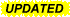# Adding and Subtracting Time For Audio Automation

Hi Everyone, I am automating audio recordings. I need to make Clip1 as long as Clip2 adding some silence to the beginning and the ending of Clip1.

Clip1 = 0:01.216
Clip2 = 0:01.234

The formula I am trying to use is: (Clip2 - Clip1)*.5 = HalfDifference

I will then add HalfDifference of silence to the beginning of the Audio Clip 1 and at the end of the audio clip so it is as long as Audio Clip 2. I can do this in my audio editing software.

The problem is, is that when I try to use this formula in the Macro it turns red. I am thinking that it might be because the macro does not recognize the format and thinks it is a string and won’t allow me to do mathematical calculations

If this is the problem, how can I change the format to just seconds and fractions of seconds so I can do calculations on it?

This does not work:

Set Variable “HalfDifference” To Calculation “(Clip2 - Clip1) * .5”

I am teaching myself how to use Keyboard Maestro and have only a little bit of programming experience.

Gratefully,

Use the Search using Regular Expression action to split the times up in to their component parts. The do the calculation to determine the difference. Then possibly convert it back into 0:. Note that these actions don't deal with more than a minute difference. The software you are using may well be fine with handling an input time of 312.567 or 0:312.567 (for five+ minutes). If not, then you will need to take the resulting value and do some more calculations to split into the minutes/seconds part.

2 Likes

Hi Peter,

Wow, this worked perfectly! Thank you!!!

If you have the time how does this code work? (\d+):([0-9.]+) for future use.

Gratefully,

Here is a JavaScript (JXA) solution.
Questions?2018-02-19 17:58 GMT-0600
• Fix error in `timeToSec()` function.

### MACRO:   Get Half of Difference using JavaScript

~~~ VER: 1.1    2018-02-19 ~~~

Get Half of Difference using JavaScript.kmmacros (3.0 KB)
Note: This Macro was uploaded in a DISABLED state. You must enable before it can be triggered.### JXA Script

``````var kmeApp = Application("Keyboard Maestro Engine");
var Clip1 = kmeApp.getvariable('Clip1');
var Clip2 = kmeApp.getvariable('Clip2');

halfDiff = precisionRound((timeToSec(Clip2) - timeToSec(Clip1)) / 2, 3);

halfDiff

//~~~~~~~~~~~ END OF MAIN SCRIPT ~~~~~~~~~~~~~~~~~

function timeToSec(pTimeStr) {
timeArr = pTimeStr.split(":")
sec     = 0;

var numElements = timeArr.length - 1;
var iPower = 0;
for (var iElem = numElements; iElem >= 0; iElem--) {
sec     += (60**iPower * Number(timeArr[iElem]))
iPower   += 1;
} // END for

return sec;
}

function precisionRound(number, precision) {
var factor = Math.pow(10, precision);
return Math.round(number * factor) / factor;
}

``````

It is a regular expression, which is a pattern matching system.

If you select Help ➤ ICU Regular Express Reference, it will show you the details the the regular expressions.

There are lots of good sites on learning regular expressions.

If you go to https://www.debuggex.com for example, you can paste that in and it shows you the break down of the regex:In the reference (above), you can find that \d will match any digit. Then + means "match one or more of the previous thing, and [0-9.] is "any digit or a literal decimal point character". () are used for bracketing (and capturing), and colon just means itself. So you have

one or more digits; followed by a literal colon; followed by one or more [digits or decimal points]

Each set of brackets can capture to a different variable, so the first capture (one or more digits) goes into ClipNMinutes variable, and the second capture (one or more [digits or decimal points]) goes into the ClipNSeconds variable.

FWIW, my above JavaScript works for any format, as long as it ends with seconds:
`h:m:s`